# Question 10 5 pts On average, a 3-V spotlight draws 0.25 A of current and generates...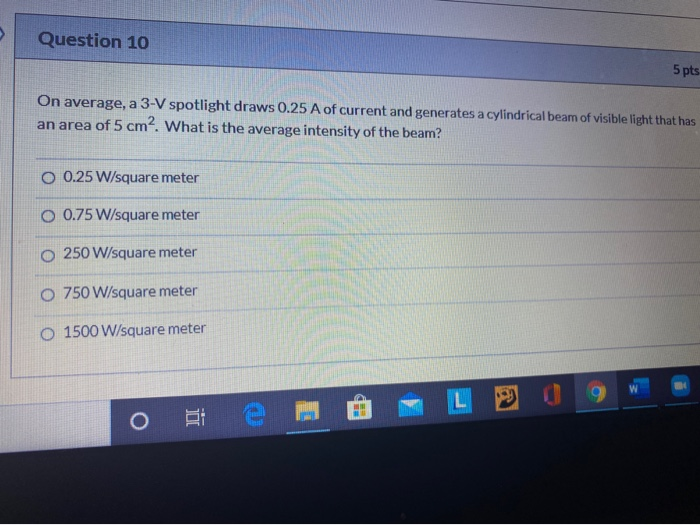Question 10 5 pts On average, a 3-V spotlight draws 0.25 A of current and generates a cylindrical beam of visible light that has an area of 5 cm2. What is the average intensity of the beam? O 0.25 W/square meter O 0.75 W/square meter o 250 W/square meter O 750 W/square meter O 1500 W/square meter L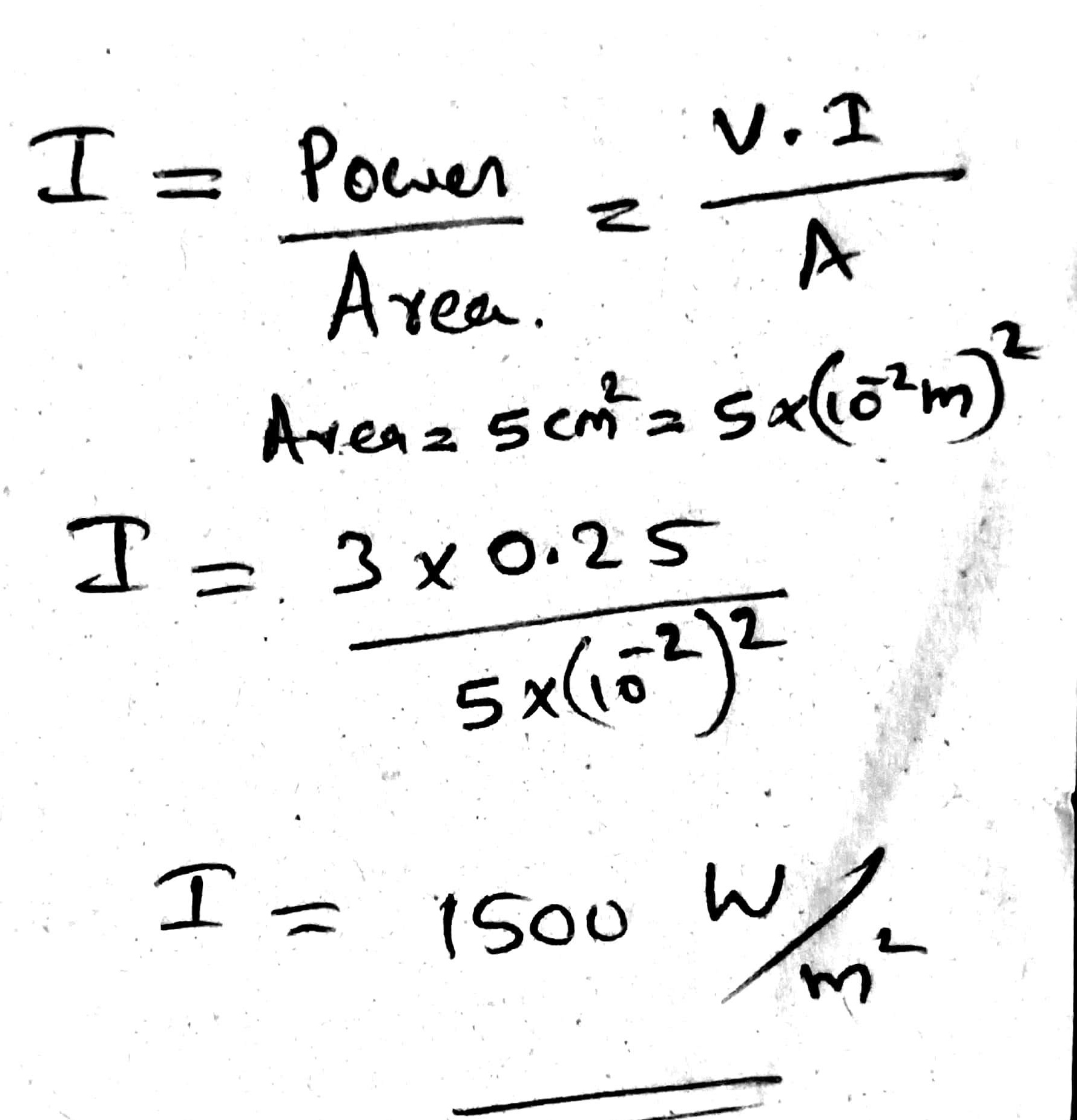##### Add Answer of: Question 10 5 pts On average, a 3-V spotlight draws 0.25 A of current and generates...
Similar Homework Help Questions
• ### Question 10 5 pts On average, a 3-V spotlight draws 0.25 A of current and generates...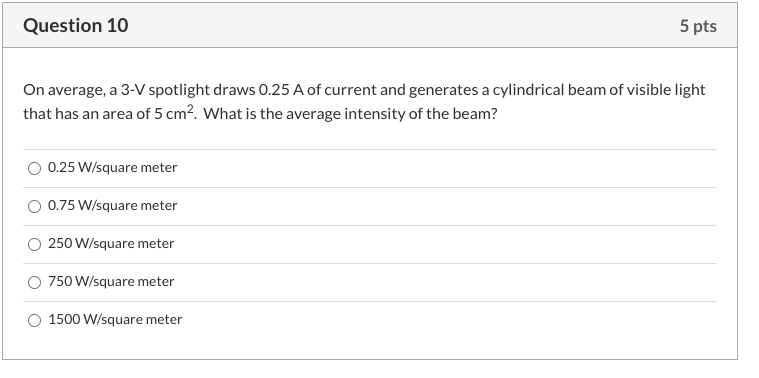Question 10 5 pts On average, a 3-V spotlight draws 0.25 A of current and generates a cylindrical beam of visible light that has an area of 5 cm? What is the average intensity of the beam? 0.25 W/square meter 0.75 W/square meter 250 W/square meter 750 W/square meter 1500 W/square meter

• ### On average, a 3-V spotlight draws 0.25 A of current and generates a cylindrical beam of...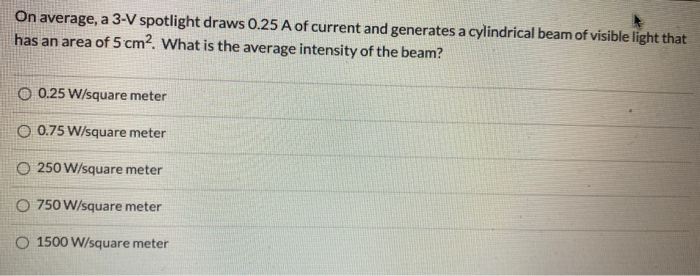On average, a 3-V spotlight draws 0.25 A of current and generates a cylindrical beam of visible light that has an area of 5 cm2. What is the average intensity of the beam? O 0.25 W/square meter O 0.75 W/square meter O 250 W/square meter O 750 W/square meter O 1500 W/square meter

• ### On average, a 3-V spotlight draws 0.25 A of current and generates a cylindrical beam of...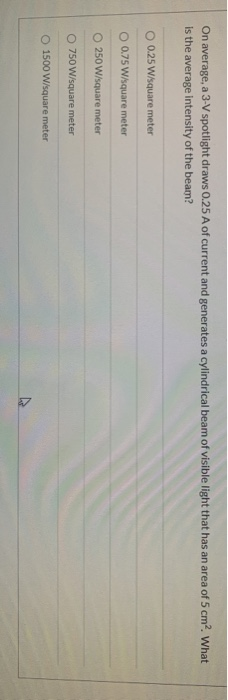On average, a 3-V spotlight draws 0.25 A of current and generates a cylindrical beam of visible light that has an area of 5 cm? What is the average intensity of the beam? O 0.25 W/square meter O 0.75 W/square meter 250 W/square meter 750 W/square meter O 1500 W/square meter

• ### Question 10 5 pts Aradio antenna emits EM waves at a frequency of 100 MHz and...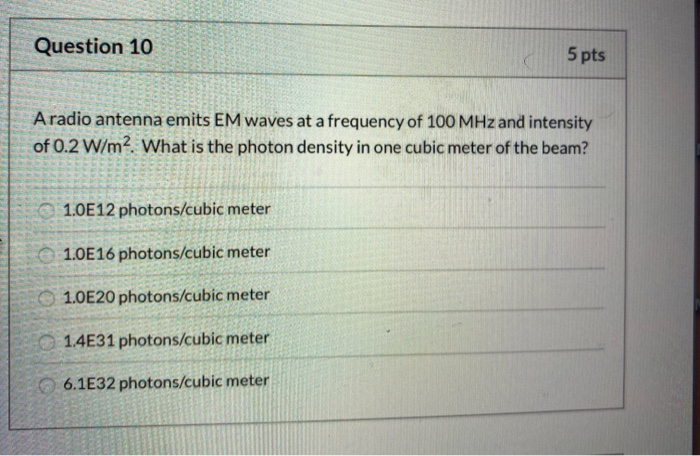Question 10 5 pts Aradio antenna emits EM waves at a frequency of 100 MHz and intensity of 0.2 W/m2. What is the photon density in one cubic meter of the beam? 1.0E12 photons/cubic meter 1.0E16 photons/cubic meter OO 1.0E20 photons/cubic meter 1.4E31 photons/cubic meter 6.1E32 photons/cubic meter Question 11 5 pts A square planar loop of coiled wire has a length of 0.25 m on a side and is rotated 60 times per second between the poles of a...

• ### Question 10 5 pts A radio antenna emits EM waves at a frequency of 100 MHz...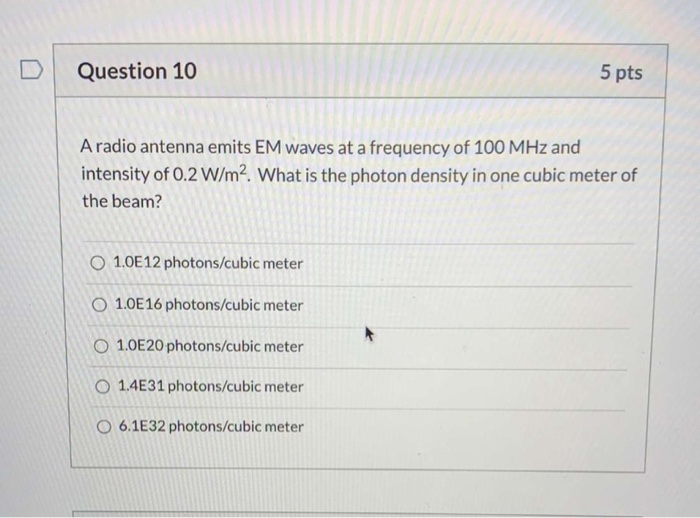Question 10 5 pts A radio antenna emits EM waves at a frequency of 100 MHz and intensity of 0.2 W/m2. What is the photon density in one cubic meter of the beam? 1.0E 12 photons/cubic meter O 1.0E 16 photons/cubic meter O 1.0E20 photons/cubic meter O 1.4E31 photons/cubic meter O 6.1E32 photons/cubic meter

• ### Question 10 5 pts A radio antenna emits EM waves at a frequency of 100 MHz...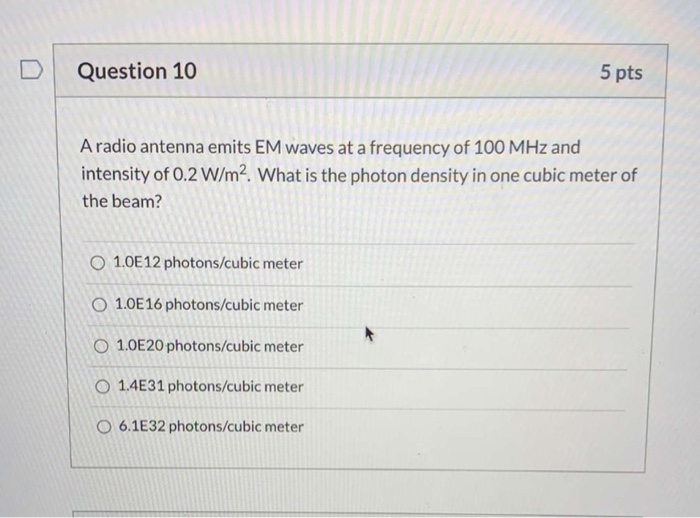Question 10 5 pts A radio antenna emits EM waves at a frequency of 100 MHz and intensity of 0.2 W/m2. What is the photon density in one cubic meter of the beam? 1.0E 12 photons/cubic meter O 1.0E 16 photons/cubic meter O 1.0E20 photons/cubic meter O 1.4E31 photons/cubic meter O 6.1E32 photons/cubic meter

• ### Question 10 5 pts A laser pointer delivers an average power of 2 mW in a...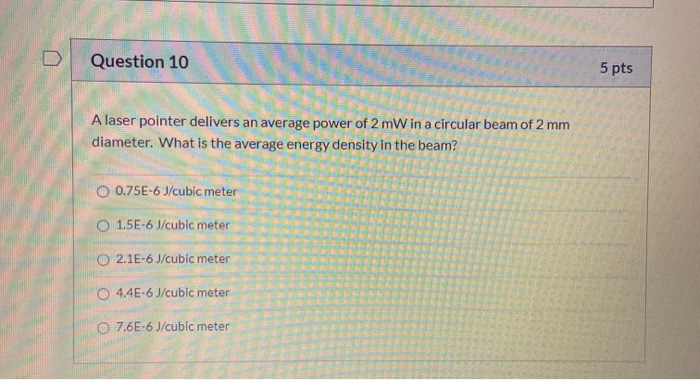Question 10 5 pts A laser pointer delivers an average power of 2 mW in a circular beam of 2 mm diameter. What is the average energy density in the beam? O 0.75E-6 J/cubic meter O 1.5E-6 J/cubic meter O 2.1E-6 J/cubic meter 44E-6 J/cubic meter 0 7.6E-6 J/cubic meter

• ### Question 10 5 pts A radio antenna emits EM waves at a frequency of 100 MHz...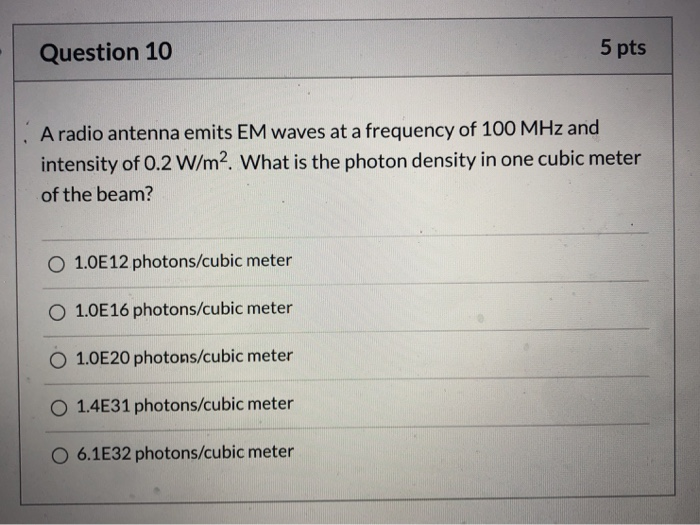Question 10 5 pts A radio antenna emits EM waves at a frequency of 100 MHz and intensity of 0.2 W/m2. What is the photon density in one cubic meter of the beam? 1.0E12 photons/cubic meter 1.0E 16 photons/cubic meter 1.0E20 photons/cubic meter O 1.4E31 photons/cubic meter O 6.1E32 photons/cubic meter

• ### Question 10 5 pts A laser pointer delivers an average power of 2 mW in a...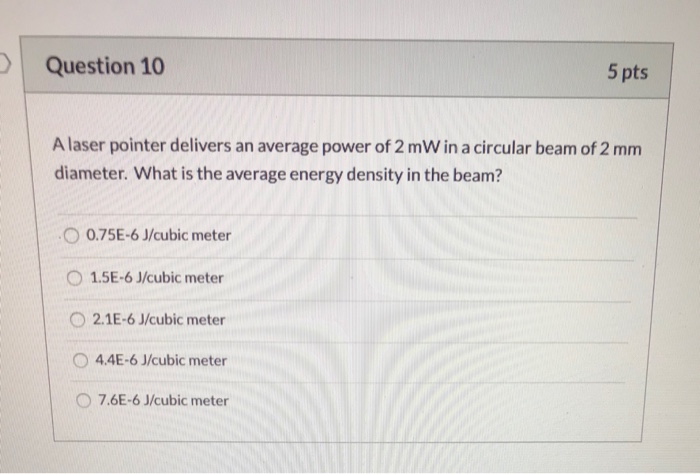Question 10 5 pts A laser pointer delivers an average power of 2 mW in a circular beam of 2 mm diameter. What is the average energy density in the beam? O 0.75E-6 J/cubic meter 1.5E-6 J/cubic meter 2.1E-6 J/cubic meter O 4.4E-6 J/cubic meter 7.6E-6 J/cubic meter

• ### Question 10 5 pts A radio antenna emits EM waves at a frequency of 100 MHz...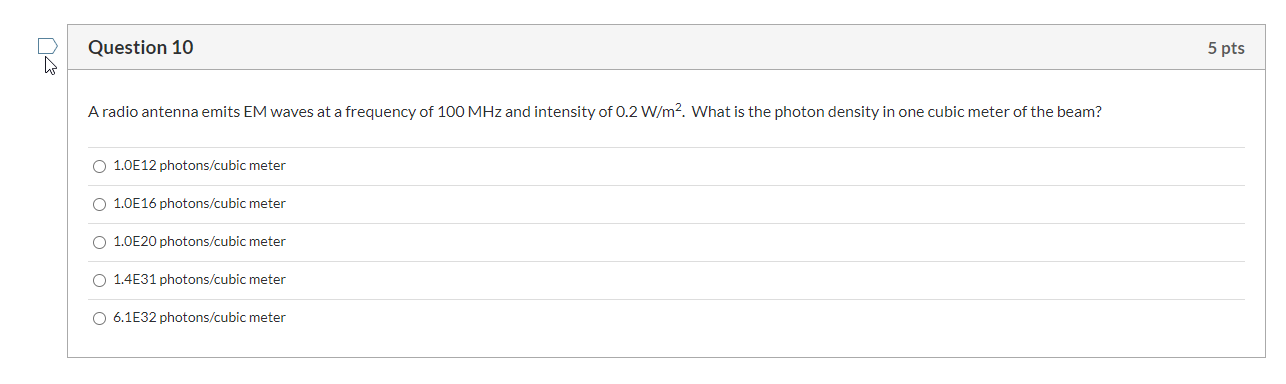Question 10 5 pts A radio antenna emits EM waves at a frequency of 100 MHz and intensity of 0.2 W/m2. What is the photon density in one cubic meter of the beam? 1.0E12 photons/cubic meter 1.0E16 photons/cubic meter 1.0E20 photons/cubic meter O 1.4E31 photons/cubic meter 06.1E32 photons/cubic meter

Free Homework App